### Chemical Reactions Class 10th Science Tamilnadu Board Solution

##### Question 1.What type of chemical reaction takes place when i) limestone is heated?ii) a magnesium ribbon is burnt in air?Answer:(i) When limestone is heated the category of chemical reraction which takes place is decomposition reaction as during this reaction the limestone decomposes to form Calcium oxide with the liberation of carbon dioxide according to the following equation : -CaCO3→ CaO + CO2↑ ( Reaction is taking place when heat is provided to limestone)(ii) When a magnesium ribbon is burnt in air the category of chemical reaction which takes place is Combination reaction. During the reaction when we burn magnesium in the presence of air Magnesium combines with oxygen from the air to form a single product namely magnesium oxide as the product. Hence, it is a combination reaction and follows the following equation: -2Mg + O2 → 2MgOQuestion 2.The pH values of certain familiar substances are given below: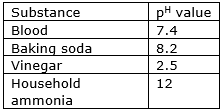Analyse the data in the table and answer the following questions:i) Which substances are acidic in nature?ii) Which substances are basic in nature?Answer:(i) A substance is treated as acidic in nature when its pH falls below 7 i.e pH < 7 in the table given in the question the only substance whose pH <7 is Vinegar. Hence, Vinegar is acidic in nature.(ii) A substance is treated as basic in nature when its pH is above 7 i.e pH > 7 in the table given in the question the substances whose pH > 7 are Blood(slightly basic), Baking soda and Household ammonia. Hence, Blood, Baking soda and Household ammonia are basic in nature.Question 3.Why does the colour of copper sulphate change when an iron nail is kept in it? Justify your answer.Answer:According to principle a more reactive element can displace a less reactive element from it’s compound. Iron is more reactive than copper hence when we place iron nails in a solution of copper sulphate the blue colour of the copper sulphate solution changes to green colour and the portion of the iron nail which was dip in the solution acquires a brown colour. This is because iron being more reactive than copper displaces copper from the copper sulphate solution and form ferrous sulphate and the displaced copper gets deposited on the portion of the nail which is dipped in the solution giving a brown colour. The following reaction takes place : -CuSO4 + Fe → FeSO4 + CuQuestion 4.The hydroxide ion concentration of a solution is 1.0 x 10 –8M. What is the pH of the solution?Answer:Given:The hydroxide ion concentration of a solution=[OH-]=1.0 x 10-8MWe know that : -pOH = - log10 [OH-]⇒ pOH = - log10[1.0 x 10-8]⇒ pOH = -[ log101.0 + log1010-8 ]Now, log101.0 = 0⇒ pOH = -[0 + (-8) log1010 ]Now, log1010 = 1⇒ pOH = -[0-8 ]⇒ pOH = -[-8]⇒ pOH = 8According to relation : -PH + pOH = 14⇒ PH = 14 - pOH⇒ PH = 14 – 8⇒ PH = 6Hence, pH of the solution is 6.Question 5.Equal lengths of magnesium ribbons are taken in test tubes A and B. Hydrochloric acid is added to test tube A, while acetic acid is added to test tube B. The amount and concentration taken for both the acids are same. In which test tube does the reaction occur more vigourously and why?Answer:We know that the nature of the reactant influences the rate of reaction. Out of Hydrochloric acid and acetic acid Hydrochloric acid is more reactive than acetic acid due to which in the test tube containing Hydrochloric acid and ribbon the rate of reaction would be much faster than in the test tube containing acetic acid and ribbon. Hence in the test tube A the reaction will occur more vigourously due to the reason mentioned above.Question 6.Two acids ‘A’ and ‘B’ were kept in beakers. Acid ‘A’ undergoes partial dissociation in water, whereas acid ‘B’ undergoes complete dissociation in water.i) Of the two acids ‘A’ and ‘B’ , which is weak acid and which is strong acid?ii) What is a weak acid?iii) What is a strong acid?iv) Give one example each.Answer:(i) We know that a strong acid undergoes complete dissociation in water whereas a weak acid undergoes only partial dissociation in water. In the case as mentioned in the question the acid ‘A’ undergoes partial dissociation in water, whereas acid ‘B’ undergoes complete dissociation in water. Hence, acid A is a weak acid and acid B is a comparatively strong acid.(ii) Weak acids are those chemical species which undergoes only partial dissociation in water and is not able to dissociate completely to release all of its H+ ions.(iii) Strong acids are those chemical species which undergoes complete dissociation in water and are able to dissociate completely to release all of its H+ ions.(iv) An example of weak acid is – acetic acid.An example of a strong acid is – Sulphuric acidQuestion 7.Observe the given chemical change and answer the following: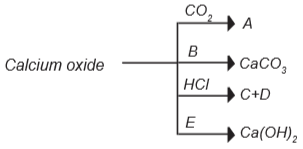i) Identify ‘A’ and ‘B’.ii) Write the commercial name of calcium hydroxide.iii) Identify products ‘C’ and 'D' , when HCl is allowed to react with calcium oxide.iv) Say whether calcium oxide is acidic or basic.Answer:(i) A is Calcium carbonate (CaCO3 ) and B is carbon dioxide (CO2). Both of these are obtained from the following reaction : -CaO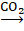CaCO3(ii) The commercial name of calcium hydroxide is slaked lime.(iii) The products ‘C’ and 'D' , when HCl is allowed to react with calcium oxide are Calcium chloride(CaCl2) and water(H2O).(iv) Calcium oxide is basic, it could be understood by its reaction with an acid as in the above case with hydrochloric acid. With hydrochloric acid it reacts to form a salt(Calcium chloride(CaCl2)) and water in the same way as a base reacts with an acid to form salt and water. Hence calcium oxide is basicQuestion 8.Take copper nitrate in a test tube and heat it over the flame.i) What is the colour of cupric nitrate?ii) What do you observe?iii) Name the type of reaction that takes place.iv) Write the balanced equation.Answer:(i) The colour of cupric nitrate is deep blue green colour.(ii) When copper nitrate is heated in a test tube it loses the water molecule or the water of crystallisation present in it and then when it is further heated it decomposes to give Copper oxide, nitrogen dioxide and oxygen. It would be observed during the reaction that a reddish brown colour gas(nitrogen dioxide) is evolved.(iii) The above reaction is a type of decomposition reaction.(iv) The balanced equation is : -2Cu(NO3)2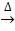2CuO + 4NO2↑ + O2↑Question 9.Identify the wrong statements and correct them.i) Sodium benzoate is used in food preservative.ii) Nitric acid is not used as fertilizer in agriculture.iii) Sulphuric acid is called the king of chemicals.iv) The PH of acid is greater than 7.v) Acetic acid is used in aerated drinks.Answer:(i) Sodium benzoate is used in food preservative. This statement is correct.(ii) Nitric acid is not used as fertilizer in agriculture. This is a wrong statement and the correct statement is Nitric acid is used as fertilizer not directly but nitric acid id used in the production of ammonium nitrate which is then used as a fertilizer.(iii) Sulphuric acid is called the king of chemicals. This is a is correct statement.(iv) The PH of acid is greater than 7. This is a wrong statement and the correct statement is The PH of acid is less than 7.(v) Acetic acid is used in aerated drinks. This is a wrong statement and the correct statement is Carbonic acid is used in aerated drinks.Question 10.Redox reactions are reactions during which electron transfer takes place. Heremagnesium atom transfers two electrons one each to the two chlorine atoms.i) What are the products of this reaction?ii) Write the balanced equation for the complete reaction.iii) Which element is being oxidized?iv) Which element is being reduced?v) Write the reduction part of the reaction.Answer:(i) The product of this reaction is – MgCl2(ii) The balanced equation for the complete reaction is : -Mg(s) + Cl2(g) → MgCl2(s)(iii) In this reaction magnesium is the element which is being oxidised as it is losing two electrons and gets converted to a stable cation Mg2+.(iv) In this reaction chlorine is the element which is getting reduced as the two chlorine atoms accepts one electron each and gets convert into anion Cl-.(v) Reduction part of the reaction is : -2Cl + 2e-→ 2Cl-Question 11.Suggest a reason for each observation given below.i) In fireworks, powdered magnesium is used rather than magnesium ribbon.ii) Zinc and dilute H2SO4 react much more quickly when a few drops of copper sulphate solutions are added.iii) The reaction between magnesium carbonate and dilute hydrochloric acid speedsup when some concentrated HCl is added.Answer:(i) We know that the rate of a reaction depends on the surface area available for the reaction to take place. The more the surface area more will be the rate of the reaction. Powdered magnesium is having more surface area than magnesium ribbon hence powdered magnesium is used rather than magnesium ribbon in fireworks so that the rate of the reaction is more.(ii) We know that a catalyst is a substance which increases the rate of a reaction without itself getting reacted. In the reaction of Zinc and dilute H2SO4 copper sulphate solution acts as a catalyst and increases the rate of the reaction.(iii) The rate of a chemical reaction increases as the concentration of the reactants increases hence the reaction between magnesium carbonate and dilute hydrochloric acid speeds up when some concentrated HCl is added as by doing so we are increasing the concentration of the reactants.Question 12.Sodium hydroxide and hydrochloric acid react as shown in this equation.NaOH + HCl → NaCl + H2O(aq) (aq) (aq) (l)i) Which type of chemical reaction is this?ii) The reaction is exothermic. Explain what that means.iii) Differentiate exothermic reaction and endothermic reaction.iv) What happens to the temperature of the solution as the chemicals react?Answer:(i) This is a type of neutralisation reaction in which an acid reacts with base to form salt and water.(ii) It is said that the reaction is exothermic which means that the reaction proceeds with evolution of heat energy.(iii)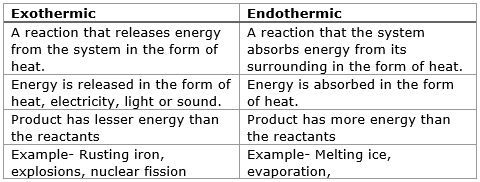(iv) The temperature of the solution increases as the chemicals reacts.Question 13.Take two conical flasks. Label them as I and II. Take a small amount of copper sulphate solution in the first conical flask. Take a small amount of granulated zinc in the second conical flask. Allow the copper sulphate solution to react with the zinc.i) Name the type of reaction.ii) Say whether the metal zinc is more reactive or less reactive.iii) Write the complete and balanced reaction.iv) Say whether this change is reversible or irreversible.Answer:(i) This reaction comes under the category of displacement reactions.(ii) A metal is said to be more reactive if it is able to displace another metal from its compound or salt solution. Here, Zinc had displaced Cu from the compound of Copper Sulphate. Hence, Zinc is more reactive.(iii) The complete and balanced reaction equation is : -Zn(s) + CuSO4(aq) → Cu(s) + ZnSO4 (aq)(iv) This change is irreversible as we would not be able to reverse the reaction to get back the reactants from the products under similar conditions.Question 14.Relate the information given in all the four columns of the table.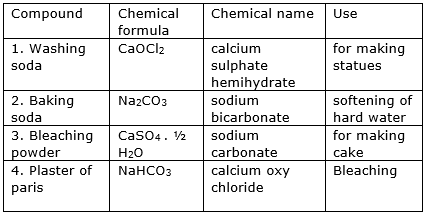Answer: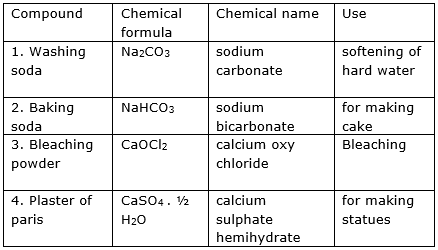Question 15.When lead powder is added to copper chloride solution, a displacement reaction occurs and solid copper is formed.i) Write the equation for the reaction.ii) Why does the displacement reaction occur?Answer:(i) The equation for the reaction is : -Pb(s) + CuCl2(aq) → PbCl2(aq) + Cu(s)(ii) The displacement reaction occurs because Lead is more reactive then Copper and hence it is able to displace Copper from its salt solution and form compound.Question 16.When zinc and copper (II) sulphate are heated together, the following redox reaction occurs:Zn + CuSO4→ ZnSO4 + Cu(s) (aq) (aq) (s)i) What does the word redox stand for?ii) Show how electrons are transferred in the reaction.iii) Write the ionic equation for the redox reaction.Answer:(i) Redox stands for those reactions in which oxidation and reduction takes place simultaneously.(ii) The scheme of elctron transfer in this reaction could be understood from the following equation : -Zn → Zn2+ + 2e-The stable ionic species the force of attraction between which forms the compound CuSO4 are Cu2+ and SO42-.Now the electrons which are liberated by Zn are accepted by Cu2+ which is then displaced from the compound to form the elemental copper and the sulphate anion left (SO42-) then combines with Zn2+ already present in the solution to form ZnSO4.(iii) The ionic equation for the redox reaction is : -Zn(s) + Cu2+SO42-→ Zn2+ SO42- + Cu(s)Question 17.If a substance gains oxygen during a reaction, it is being oxidized. If it loses oxygen, it is being reduced. Oxidation and Reduction always take place together, so that if one substance is oxidized, another is reduced. Using this idea, say which substance is oxidised and which substance is reduced in each reaction.i) Mg + O2→ 2MgO(s) (g) (s)ii) ZnO + C →Zn + COiii) Fe2 O3+ 3CO →2Fe + 3CO2iv) Cr2O3 + 2Al → + 2Cr + Al2O3Answer:(i) Mg + O2→ 2MgOAs oxidation is the process of addition of oxygen and in the above reaction which is a combination reaction oxygen is being added to magnesium to form magnesium oxide. Hence, Magneium is oxidised in the above reaction.(ii) ZnO + C →Zn + COIn the above reaction which could be treated as a single displacement reaction oxygen is being removed from the compound Zinc Oxide i.e. Zinc is losing the oxygen atom and Carbon is gaining the oxygen atom. Hence, Zinc is oxidized and Carbon is reduced in the above reaction.(iii) Fe2 O3+ 3CO →2Fe + 3CO2In the above reaction, Iron is losing the oxygen atom and Carbon is gaining the oxygen atom. As oxidation processes in which a substance gains oxygen during a reaction and reduction is a process in which a substance losses oxygen during a reaction. Hence, in the above reaction Iron is reduced and Carbon is oxidised.(iv) Cr2O3 + 2Al → + 2Cr + Al2O3In the above reaction Chromium is losing oxygen and Aluminium is gaining the oxygen atoms. Hence, Chromium is being reduced and Aluminium is oxidised.Question 18.The hydrogen ion concentration of a solution is 1 X 10 -8 Mi) What is the PH of the solution?ii) What is the POH of the solution?iii) Is the given solution, acidic or basic?.Answer:(i) We are given that the hydrogen ion concentration of a solution is = [H+] = 1 X 10-8 MWe know that : -pH = - log10 [H+]⇒ pH = - log10 [1 X 10-8 ]⇒ pH = -[ log101 + log1010-8 ]Now, log101 = 0⇒ pH = -[0 + (-8) log1010 ]Now, log1010 = 1⇒ pH = -[0-8 ]⇒ pH = -[-8]⇒ pH = 8Hence, the pH of the solution is 8.(ii) According to relation we have : -PH + pOH = 14⇒ 8 + pOH = 14⇒ pOH = 14 – 8⇒ pOH = 6Hence, the pOH of the solution is 6.(iii) As the pH of the solution is 8 which is greater than 7. Hence, the given solution is basic.

PDF FILE TO YOUR EMAIL IMMEDIATELY PURCHASE NOTES & PAPER SOLUTION. @ Rs. 50/- each (GST extra)

HINDI ENTIRE PAPER SOLUTION

MARATHI PAPER SOLUTION

SSC MATHS I PAPER SOLUTION

SSC MATHS II PAPER SOLUTION

SSC SCIENCE I PAPER SOLUTION

SSC SCIENCE II PAPER SOLUTION

SSC ENGLISH PAPER SOLUTION

SSC & HSC ENGLISH WRITING SKILL

HSC ACCOUNTS NOTES

HSC OCM NOTES

HSC ECONOMICS NOTES

HSC SECRETARIAL PRACTICE NOTES

2019 Board Paper Solution

HSC ENGLISH SET A 2019 21st February, 2019

HSC ENGLISH SET B 2019 21st February, 2019

HSC ENGLISH SET C 2019 21st February, 2019

HSC ENGLISH SET D 2019 21st February, 2019

SECRETARIAL PRACTICE (S.P) 2019 25th February, 2019

HSC XII PHYSICS 2019 25th February, 2019

CHEMISTRY XII HSC SOLUTION 27th, February, 2019

OCM PAPER SOLUTION 2019 27th, February, 2019

HSC MATHS PAPER SOLUTION COMMERCE, 2nd March, 2019

HSC MATHS PAPER SOLUTION SCIENCE 2nd, March, 2019

SSC ENGLISH STD 10 5TH MARCH, 2019.

HSC XII ACCOUNTS 2019 6th March, 2019

HSC XII BIOLOGY 2019 6TH March, 2019

HSC XII ECONOMICS 9Th March 2019

SSC Maths I March 2019 Solution 10th Standard11th, March, 2019

SSC MATHS II MARCH 2019 SOLUTION 10TH STD.13th March, 2019

SSC SCIENCE I MARCH 2019 SOLUTION 10TH STD. 15th March, 2019.

SSC SCIENCE II MARCH 2019 SOLUTION 10TH STD. 18th March, 2019.

SSC SOCIAL SCIENCE I MARCH 2019 SOLUTION20th March, 2019

SSC SOCIAL SCIENCE II MARCH 2019 SOLUTION, 22nd March, 2019

XII CBSE - BOARD - MARCH - 2019 ENGLISH - QP + SOLUTIONS, 2nd March, 2019

HSC Maharashtra Board Papers 2020

(Std 12th English Medium)

HSC ECONOMICS MARCH 2020

HSC OCM MARCH 2020

HSC ACCOUNTS MARCH 2020

HSC S.P. MARCH 2020

HSC ENGLISH MARCH 2020

HSC HINDI MARCH 2020

HSC MARATHI MARCH 2020

HSC MATHS MARCH 2020

SSC Maharashtra Board Papers 2020

(Std 10th English Medium)

English MARCH 2020

HindI MARCH 2020

Hindi (Composite) MARCH 2020

Marathi MARCH 2020

Mathematics (Paper 1) MARCH 2020

Mathematics (Paper 2) MARCH 2020

Sanskrit MARCH 2020

Sanskrit (Composite) MARCH 2020

Science (Paper 1) MARCH 2020

Science (Paper 2)

MUST REMEMBER THINGS on the day of Exam

Are you prepared? for English Grammar in Board Exam.

Paper Presentation In Board Exam

How to Score Good Marks in SSC Board Exams

Tips To Score More Than 90% Marks In 12th Board Exam

How to write English exams?

How to prepare for board exam when less time is left

How to memorise what you learn for board exam

No. 1 Simple Hack, you can try out, in preparing for Board Exam

How to Study for CBSE Class 10 Board Exams Subject Wise Tips?

JEE Main 2020 Registration Process – Exam Pattern & Important Dates

NEET UG 2020 Registration Process Exam Pattern & Important Dates

How can One Prepare for two Competitive Exams at the same time?

8 Proven Tips to Handle Anxiety before Exams!